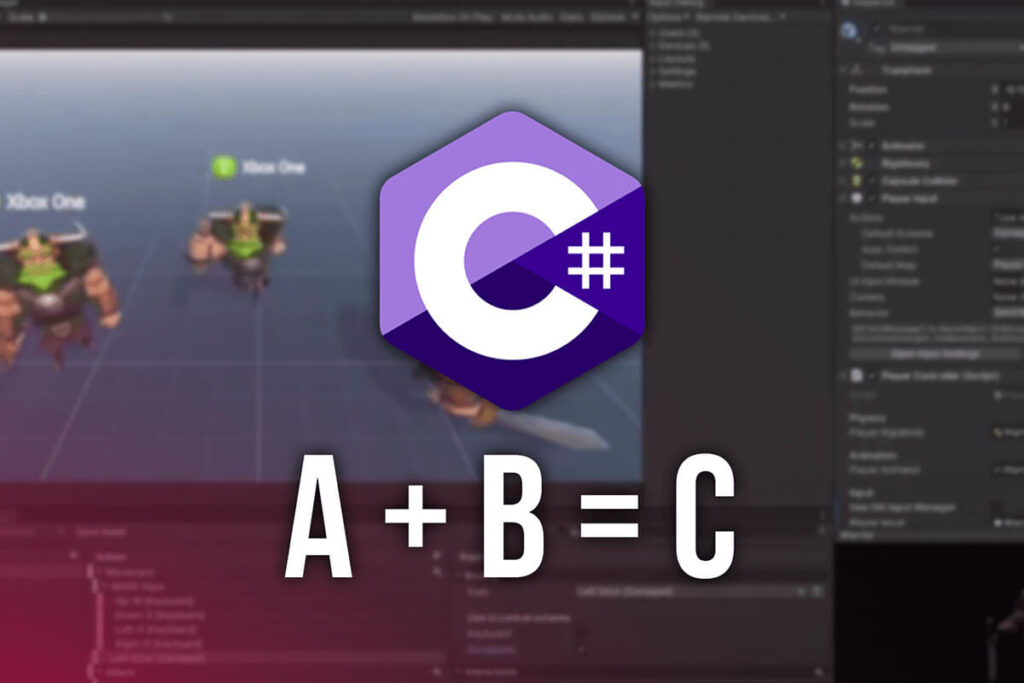# C# Programming With Unity – Math Operations With Variables

Level: Beginner
Version: 2020.3.1 LTS

Help Others Learn Game Development

The scariest part of programming is math and complex physics formulas, well, this is what the majority of people believe.

But in fact this is not true at all. You don’t have to be a math expert or a Nobel price physicist in order to learn how to code and make games. You just need to know the basic math operations which are addition, subtraction, multiplication and division. And that’s it.

Now you are looking at me all confused and saying to yourself this dude is crazy as a….. a crazy person. I kid you not, everything you need to know that is connected to math in any way, shape, or form is what I mentioned above.

All other complex calculations like finding an angle between two points, all of that is already predefined and we don’t need to code that ourselves, but we will see examples of that when the time comes, because now, we are going to jump at the topic at hand and start with addition.

Everything that we are going to talk about from now on is in some way connected to the variables that we learned about in the previous post. So when it comes to math operations we can perform them on the number variables like floats, doubles, and integers.

In the Start function between the opening {} in your script, you can declare 2 float variables called a and b:

```				```
void Start()
{
float a = 2.3f;
float b = 3.5f;

}
```
```

And we can create a third float variable c that will be equal to the sum of a and b:

```				```
float c = a + b;
```
```

This line of code will calculate the sum of a and b and set that value to the new c variable. And we can verify this by printing this to the console by adding the line of code below:

```				```
Debug.Log("The value of c is: " + c);
```
```

Now your code should look like this:

```				```
void Start()
{
float a = 2.3f;
float b = 3.5f;

float c = a + b;

Debug.Log("The value of c is: " + c);

}
```
```

The Debug.Log is used to print to the console tab in the editor, it takes a string as parameter hence the “” that you see between the parenthesis. The c variable is a float type variable that’s why you can’t write something like

```				```
Debug.Log("The value of c is:  + c");
```
```

Because this line of code will print everything that you see between the “” in parenthesis.

But when we use + with a string and we provide another variable, be that a float, int, bool or even a string, it is going to form a new string by using what is before and what is after the + sign. This is called string concatenation.

To verify this we need to save our script by holding CRTL + S or CMD + S on Mac OS, and then attach our script to a game object that is in the Hierarchy panel.

This can be any game object, it can be the Camera, or you can create a new game object by Right Click-> Create Empty: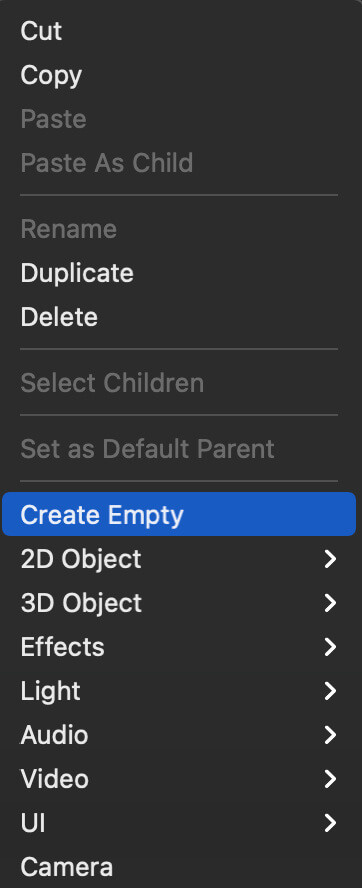You can double click on the game object’s name and rename it how ever you want: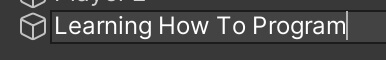Now, you need to click on that game object in the Hierarchy, and drag the script directly on the game object, or drag it on the Inspector tab where the properties and other components attached to that game object are exposed: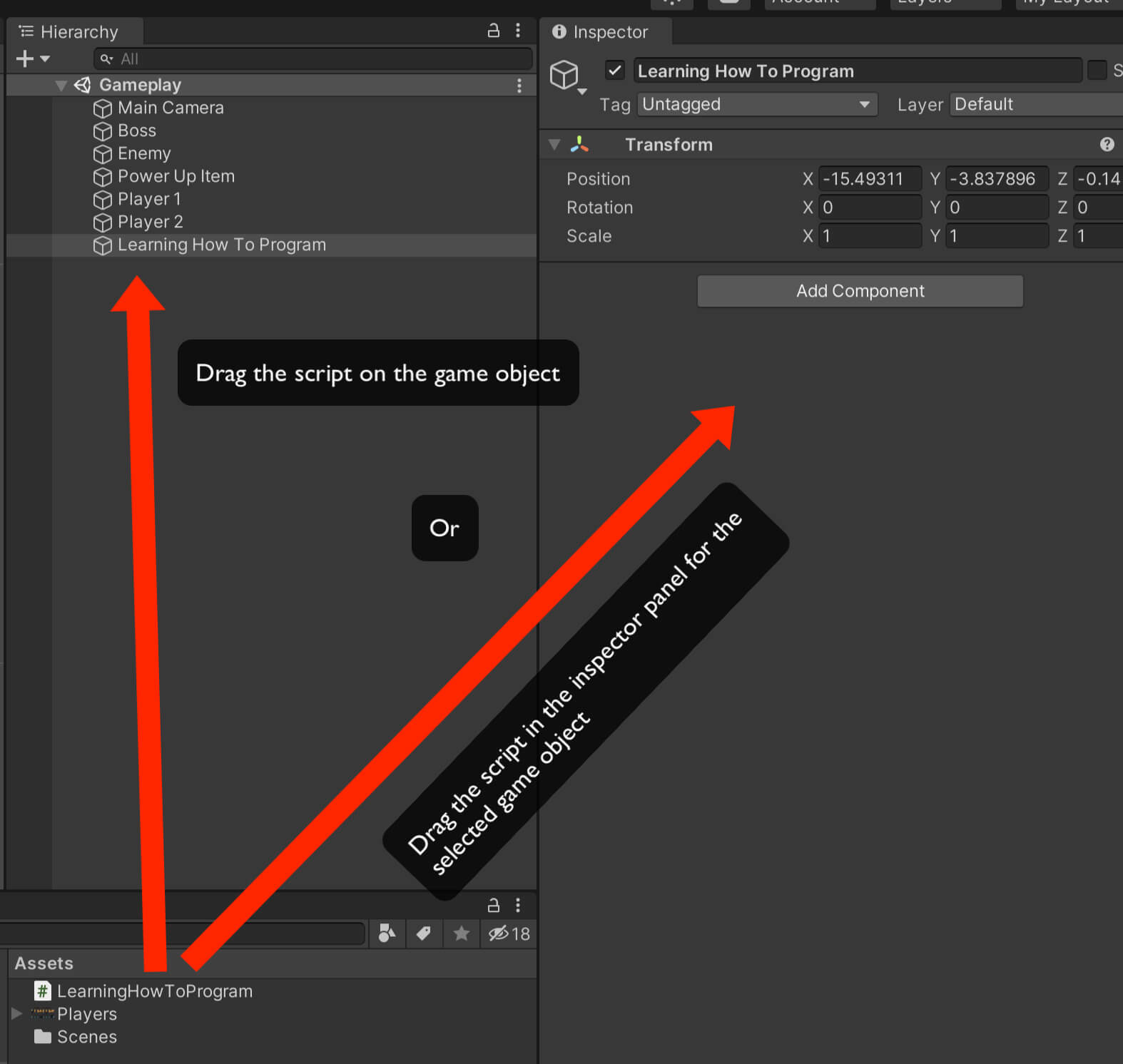There is also a third way how you can attach a script or any other component on the game object.

Select the game object in the Hierarchy panel, and in the Inspector panel click on the “Add Component” button and from the search list either locate the component you want to attach, or type the name of the component in the search bar and attach it: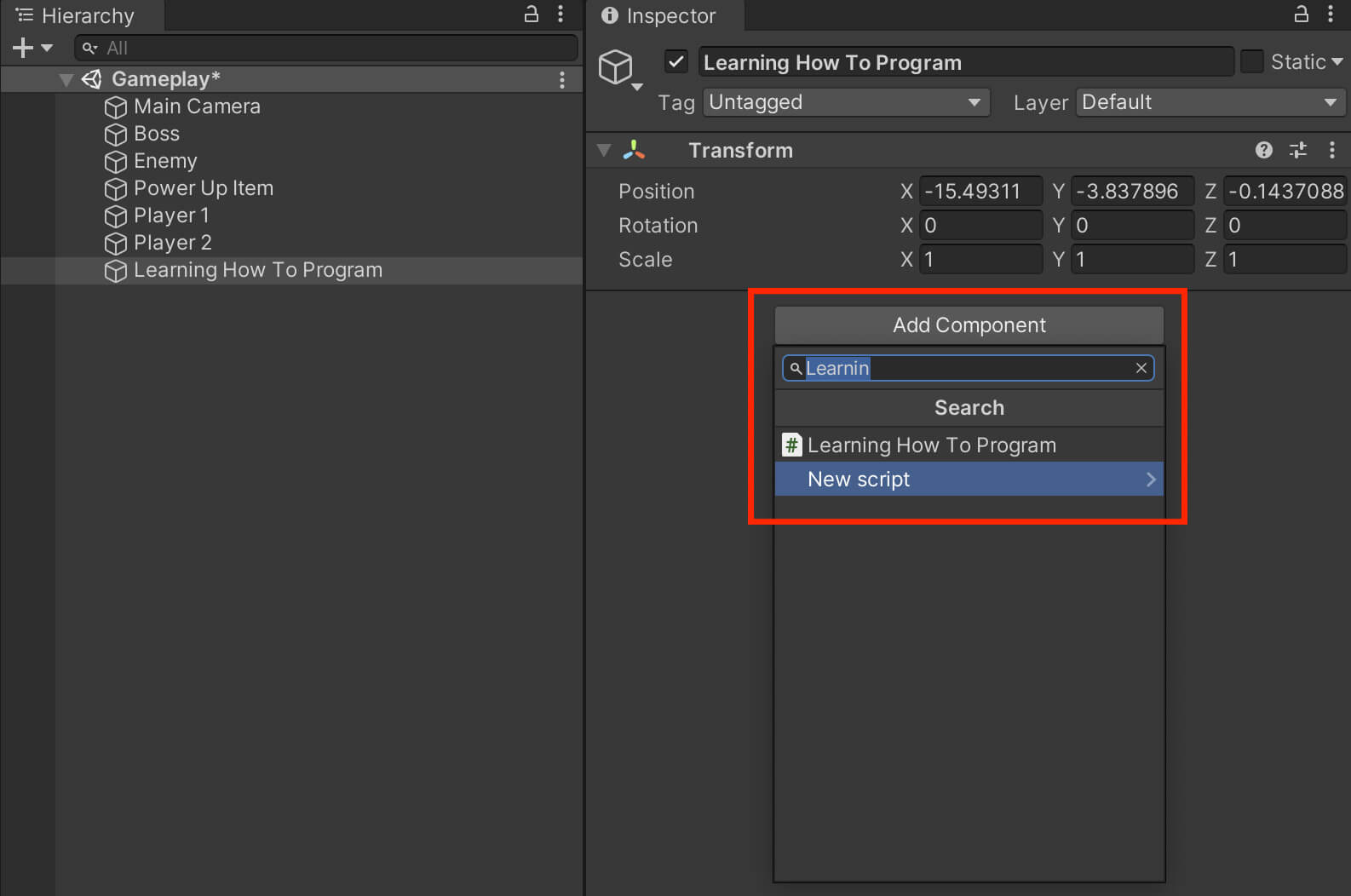When you do that successfully the script will be attached on the game object as a component and you will see this: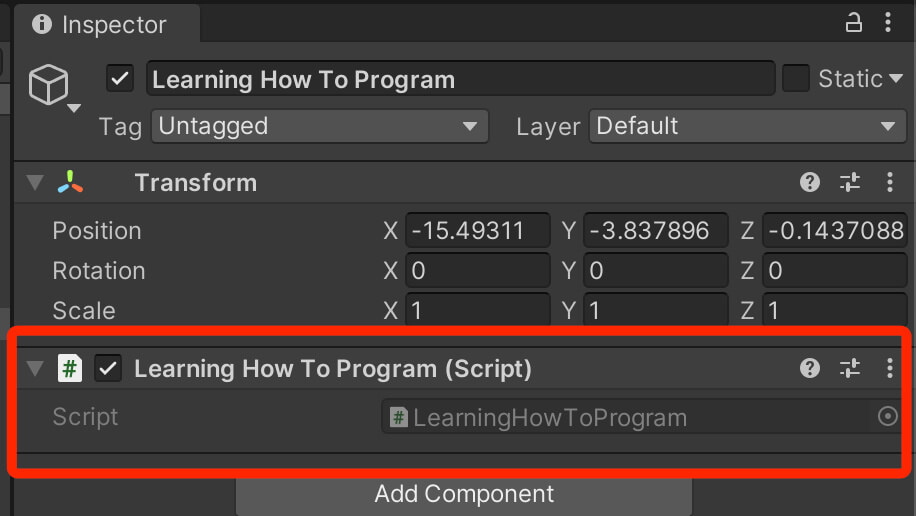And this is how we attach scripts on game objects.

Other components such as AudioSource, Rigidbody, Colliders and so on, we attach by click the “Add Component” button and filtering for the desired component.

So from now on when I say attach a specific component on a game object, you will do it the same way we attached the script now.

Now that we have the script attached and we wrote all the lines of code in the Start function, we can press the play button in the editor to run the game: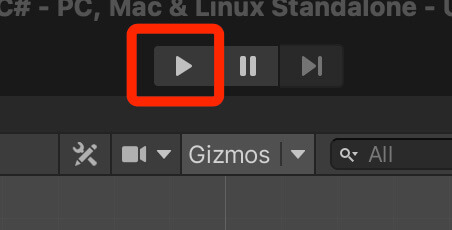This is how you run games in Unity to see and test the changes you made to the game.

For this example we are going to check out the Console tab and see the result that is printed by calculating the two floats we created previously: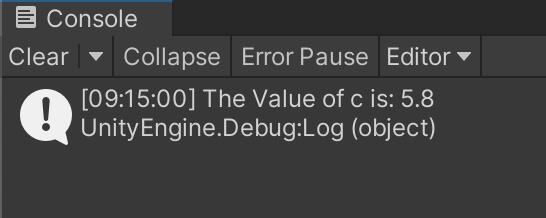As you can see the value that is printed is 5.8 which is the calculation of our floats a and b who have values 2.3 and 3.5 respectively.

### Subtraction

Similarly we can calculate the subtraction of the two variables by writing:

```				```
float d = b - a;
Debug.Log("The value of d is: " + d);
```
```

Make sure that you save your script every time you write new lines of code so that the changes you made will take affect. Press the play button and you will see the new result printed in the Console: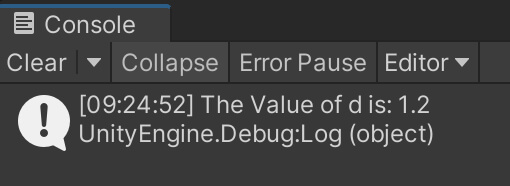I subtracted a from b because b has a value of 3.5 and a has a value of 1.2, that’s why we have 1.2 as a result, but you can also subtract b from a and the result will be a negative value. Just change the previous float d declaration to:

```				```
float d = a - b;
```
```

and run the game. This is the new result printed in the Console: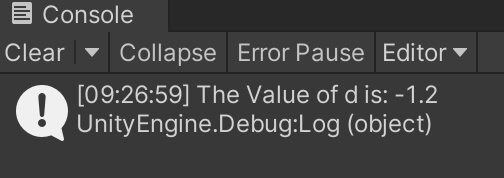The value is negative as I already mentioned, which means that your result can be a negative or a positive value which is very useful for game development and we will see that when we start building games.

### Multiplication And Division

Now the same way we calculated addition and subtraction, we can calculate the multiplication and division. Delete the previous lines of code and write the following ones:

```				```
float a = 5f;
float b = 2f;

float c = a * b;
float d = a / b;

Debug.Log("The value of c is: " + c);
Debug.Log("The value of d is: " + d);
```
```

Run the game and check out the result in the Console: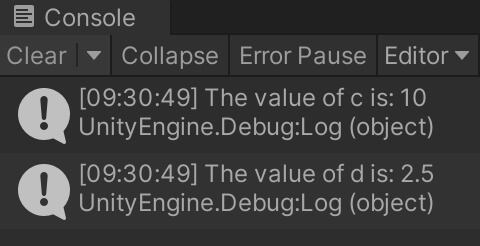This is the scary math in programming that everyone is afraid of, it’s as simple as writing 1 + 1 🙂

You can repeat this whole process with integers. Just replace the previous lines of code with the new ones:

```				```
int a = 6;
int b = 2;

int c = a + b;
int d = a - b;

int f = a * b;
int g = a / b;

Debug.Log("The value of c is: " + c);
Debug.Log("The value of d is: " + d);

Debug.Log("The value of f is: " + f);
Debug.Log("The value of g is: " + g);
```
```

Run the game and check out the Console: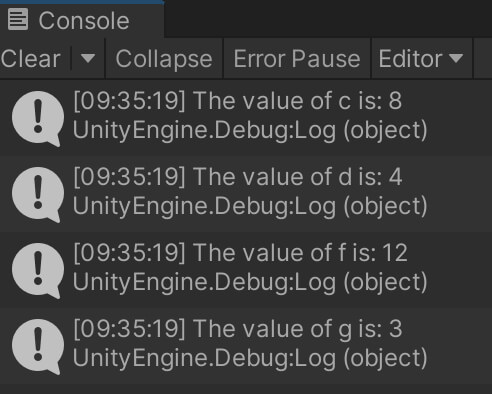### Casting

One thing to note here is that depending on the value you want to calculate you might use one variable over the other. For example, integers are whole numbers, so you can’t calculate decimal point values. If you type

```				```
int a = 5;
int b = 2;
int c = a / b;
Debug.Log("The value of c is: " + c);
```
```

You will see that the value of c is 2: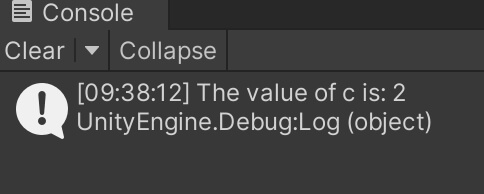We know that 5 divided by 2 is 2.5, but since integers are whole numbers they can’t store decimal point values, and if you need to calculate decimal point numbers then you will use floats for that purpose.

Similarly, if you want to calculate whole numbers with floats you are not able to do that except with casting.

This basically means that you will convert a float into an integer and the way this works is that you will add (int) in front of a float value that you are using in the calculation. For example:

```				```
float a = 5;
float b = 2;
int c = (int)a * (int)b;
Debug.Log("The value of c is: " + c);
```
```

When you run the game this is the value printed in the Console: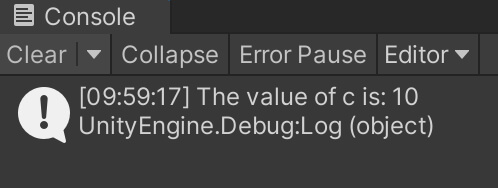If you try to calculate these values without using (int) in front of float variables, you will get an error right away indicating that you can’t do that.

### Incrementing Variables

One thing that is present a lot in programming is incrementing or decrementing values by 1, this is especially used a lot when working with arrays, which we will see down the road.

In order to increment a value you just add ++ at the end of the variable. For example:

```				```
int a = 3;
a++;
```
```

The code above will create an integer a and give it a value 3, the ++ in the following line of code will increment a by 1, which means after that line of code is executed its value of a will be 4 and not 3. To make it more clear writing a++ is the same as the example below:

```				```
a = a + 1;
```
```

The code above does the exact same thing as adding ++, but you can see that using ++ is much shorter. The point is, when you use ++ it will increase the current value of the variable by 1. Similarly, if you use  – – it will decrease the value by 1:

```				```
a--;
```
```

This is the same as if we wrote:

```				```
a = a - 1;
```
```

The outcome will be that a now has a value of 2 because we decreased it by 1.

This used often in programming as I said, and we will see a lot of examples of this and talk about it again when those examples come, but I wanted to introduce you to this concept now as it is connect to math operations with variables.

And this is it. These are all the math operations that you need to know when it comes to programming.

### Where To Go From Here

In this lecture you learned about math operations with variables and you saw that math in programming is not scary as a lot of people think it is.

To continue your learning journey you can take a look at our Introduction To Functions Lecture which is the next lecture in this tutorial series.